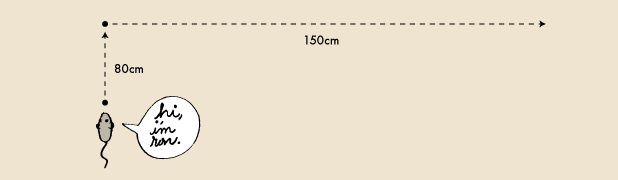# Displacement vs. Distance

Geometry Level 1Ron the Mouse travels 80 centimeters north and then 150 centimeters east.

Let $d$ be the distance traveled by Ron the Mouse and $s$ be the magnitude of the displacement of the mouse.

Find the value (in centimeters) of $d-s.$

×